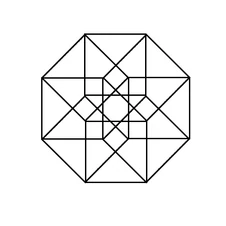2,559 PagesA tesseract is a 4-dimensional hypercube. Since the number of dimensions is a square number, the diagonal length of a tesseract is an integer - in this case, 2. Its Bowers acronym is "tes". The image shows the shadow of a rotating tesseract, keep in mind that 4D objects have 3D shadows.

### Variants

The tesseract can be seen as a cube prism, the product of a cube and a line segment. It is also the square-square duoprism, i.e. the product of two squares. It can also be seen as a square prism prism, or the product of four line segments.

## Structure and sections

The tesseract is composed of eight cubic cells. Two of these cubes line in parallel 3-D spaces, while the remaining six connect the faces of the cubes. Four cubes meet at each vertex.

In cube-first position, it is a sequence of identical cubes. In square-centered orientation, it is a square which expands to a square prism and back. When seen line-first it is a line that expands to a triangular prism, then turns to a hexagonal prism, and then back. Finally in corner first orientation, it goes through the entire tetrahedral truncation series, from point to tetrahedron to octahedron in the middle and then back.

Zeroth First Second Third Fourth Fifth Sixth Seventh Eighth Ninth Tenth
Simplex Point Line Triangle Tetrahedron Pentachoron Hexateron Heptapeton Octaexon Enneazetton Decayotton Hendecaxennon
Hypercube Point Line Square Cube Tesseract Penteract Hexeract Hepteract Octeract Enneract Dekeract
Cross Point Line Square Octahedron Hexadecachoron Pentarss Hexarss Heptarss Octarss Ennearss Decarss
Hypersphere Point Line Circle Sphere Glome Hyperglome Hexaphere Heptaphere Octaphere Enneaphere Decaphere
Community content is available under CC-BY-SA unless otherwise noted.